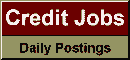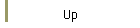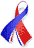DefaultRisk.com the web's biggest credit risk modeling resource.doi> search: A or B Export citation to:- HTML- Text (plain)- BibTeX- RIS- ReDIF

Sample-path Large Deviations in Credit Risk

by Vincent Leijdekker of the University of Amsterdam & ABN AMRO,
Michel Mandjes of the University of Amsterdam, and
Peter Spreij of the University of Amsterdam

September 30, 2009

Abstract: The event of large losses plays an important role in credit risk. As these large losses are typically rare, and portfolios usually consist of a large number of positions, large deviation theory is the natural tool to analyze the tail asymptotics of the probabilities involved. We first derive a sample-path large deviation principle (LDP) for the portfolio's loss process, which enables the computation of the logarithmic decay rate of the probabilities of interest. In addition, we derive exact asymptotic results for a number of specific rare-event probabilities, such as the probability of the loss process exceeding some given function.

Books Referenced in this Paper:  (what is this?)